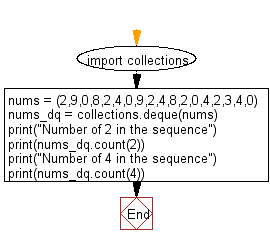﻿ Python: Count the number of times a specific element presents in a deque object - w3resource# Python: Count the number of times a specific element presents in a deque object

## Python Collections: Exercise-12 with Solution

Write a Python program to count the number of times a specific element presents in a deque object.

Sample Solution:

Python Code:

``````import collections
nums = (2,9,0,8,2,4,0,9,2,4,8,2,0,4,2,3,4,0)
nums_dq = collections.deque(nums)
print("Number of 2 in the sequence")
print(nums_dq.count(2))
print("Number of 4 in the sequence")
print(nums_dq.count(4))
```
```

Sample Output:

```Number of 2 in the sequence
5
Number of 4 in the sequence
4
```

Flowchart:## Visualize Python code execution:

The following tool visualize what the computer is doing step-by-step as it executes the said program:

Python Code Editor:

Have another way to solve this solution? Contribute your code (and comments) through Disqus.

What is the difficulty level of this exercise?

Test your Programming skills with w3resource's quiz.

﻿

## Python: Tips of the Day

The Zip() Function:

```>>> students = ('John', 'Mary', 'Mike')
>>> ages = (15, 17, 16)
>>> scores = (90, 88, 82, 17, 14)
>>> for student, age, score in zip(students, ages, scores):
...     print(f'{student}, age: {age}, score: {score}')
...
John, age: 15, score: 90
Mary, age: 17, score: 88
Mike, age: 16, score: 82
>>> zipped = zip(students, ages, scores)
>>> a, b, c = zip(*zipped)
>>> print(b)
(15, 17, 16)
```